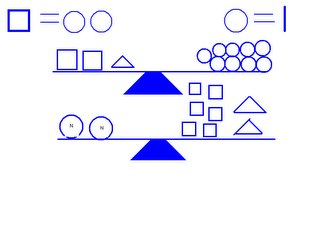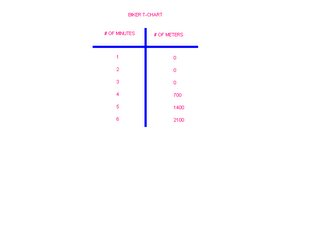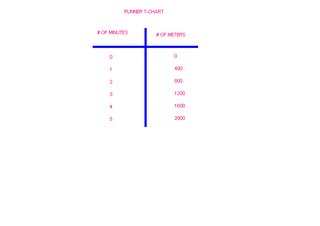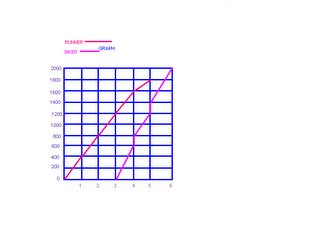## Sunday, May 28, 2006

### My Growing Post

1. In an algebraic expression you get to choose the value of the variable.
In an algebraic equation the value of the variable is given.

2.A variable is a letter that replaces a number. We use a variable in algebra because algebra is about solving the uknown and the variable is the unknown thing we have to solve.

3.To solve the second question you need to figure out what a triangle equals on the first scale. First I took away four circles (each square equals two circles), and it left me with five circles. Since there were five circles left the triangle equals five circles. To solve the second question you need to figure out the value of the two Ns. First I added the squares and triangles together. The total of the squares and triangles and it was 22 circles. Then I divided the 22 circles by 2. The answer was 11. So each N equals 11 circles.

4. .(a) 3n+4n+7=
2n+123(1)+4(1)+7=
2(1)+123+4+7=14
N= 1

b) 8n-(4+9)=1
1 8(3)-(4+9)=
1124-13=
11N= 3

(c) (8-3)n+7n+8=
4n+405n+7n+8=
4n+405(4)+7(4)+8=
4(4)+4020+28+8=56
N= 4

5.The Runner will cross the finish line first in 5 minutes. The biker will cross the finish line second in 6 minutes.

At 5/30/2006 9:25 AM,amelia_ mal said...

Hey Candy! that was Great. You are so smart! It's Amalia.

At 5/30/2006 3:35 PM,Fang_the_Barbarian said...

GREAT JOB!!!!

At 6/01/2006 3:59 PM,Mr. Reece said...

In an equation is the value of the variable given, or is the solution given and from it we can find the value of the variable?

Otherwise great job

At 6/19/2006 2:36 PM,Mr. Reece said...

you never gave an example of an equation or an expression. otherwise great job. Your mark is 27/30